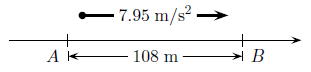# Problem: Consider a car which is traveling along a straight road with constant acceleration a. There are two checkpoints A and B which are a distance 108 m apart. The time it takes for the car to travel from A to B is 4.43 s. Find the velocity vB for the case where the acceleration is 7.95 m/s2.

###### FREE Expert Solution
80% (277 ratings)
###### Problem Details

Consider a car which is traveling along a straight road with constant acceleration a. There are two checkpoints A and B which are a distance 108 m apart. The time it takes for the car to travel from A to B is 4.43 s. Find the velocity vB for the case where the acceleration is 7.95 m/s2.